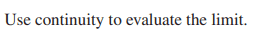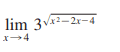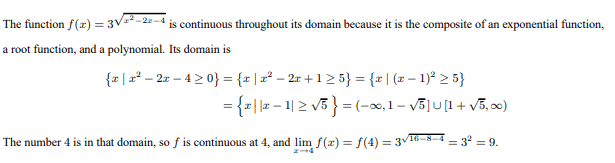# Finding domain when using continuity to evaluate a limit

• I
Callumnc1
For this problem,The solution is,However, when I tried finding the domain myself:

## { x | x - 1 ≥ \sqrt{5}} ## (Sorry, for some reason the brackets are not here)
##{ x | x - 1 ≥ -\sqrt{5}} ## and ## { x | x - 1 ≥ \sqrt{5}}##
##{x | x ≥ 1 -\sqrt{5} }## and ## { x | x ≥ \sqrt{5} + 1}##

However, I don't understand how ##x ≥ 1 -\sqrt{5}## and ##x ≥ \sqrt{5} + 1## can both be true. Because of that, I also don't understand how they got the domain.

Many thanks!

Homework Helper
Gold Member
2022 Award
However, I don't understand how ##x ≥ 1 -\sqrt{5}## and ##x ≥ \sqrt{5} + 1## can both be true.

•Callumnc1
Homework Helper
2022 Award
However, I don't understand how ##x ≥ 1 -\sqrt{5}## and ##x ≥ \sqrt{5} + 1## can both be true. Because of that, I also don't understand how they got the domain.

Many thanks!

If $|x| = 5$, are both $x = 5$ and $x = -5$ true simultaneously?

•Callumnc1
Callumnc1

I kind of remember, but it was from over a year ago now.

Many thanks!

Callumnc1
If $|x| = 5$, are both $x = 5$ and $x = -5$ true simultaneously?

Yes I believe so (if we sub the values of x into the absolute value function).

Many thanks!

Homework Helper
2022 Award

Yes I believe so (if we sub the values of x into the absolute value function).

Many thanks!

No, exactly one of those is true; $x$ cannot satisfy both $x = 5$ and $x = -5$ simultaneously, because $-5 \neq 5$. But $|x| = 5$ will be true if either of those statements is true. Thus $$\{x : |x| = 5\} = \{-5\} \cup \{5\} = \{-5,5\}.$$ How would you apply that logic to your problem?

•Callumnc1
Callumnc1
No, exactly one of those is true; $x$ cannot satisfy both $x = 5$ and $x = -5$ simultaneously, because $-5 \neq 5$. But $|x| = 5$ will be true if either of those statements is true. Thus $$\{x : |x| = 5\} = \{-5\} \cup \{5\} = \{-5,5\}.$$ How would you apply that logic to your problem?

I will do some hard thinking and get back to you.

Many thanks!

Mentor
For this problem,
View attachment 323369
View attachment 323368
The solution is,
View attachment 323370
However, when I tried finding the domain myself:

## \{ x | x - 1 ≥ \sqrt{5}\} ## (Sorry, for some reason the brackets are not here) Now fixed..
##\{ x | x - 1 ≥ -\sqrt{5}\} ## and ## \{ x | x - 1 ≥ \sqrt{5}\}##
##\{x | x ≥ 1 -\sqrt{5} \}## and ## \{ x | x ≥ \sqrt{5} + 1\}##

However, I don't understand how ##x ≥ 1 -\sqrt{5}## and ##x ≥ \sqrt{5} + 1## can both be true. Because of that, I also don't understand how they got the domain.
1. The reason the braces disappeared is that they are special characters in TeX that are used for multiple purposes (e.g., exponents, fractions, subscripts, etc.). If you need to display a left or right brace, precede it with a slash. That's what I did for the brace pairs in what you wrote above.
2. As already mentioned, the author did not write ##x ≥ 1 -\sqrt{5}## and ##x ≥ \sqrt{5} + 1##. The symbol they used was ##\cup## or union, which corresponds to "or" not "and."

•Callumnc1
Callumnc1
1. The reason the braces disappeared is that they are special characters in TeX that are used for multiple purposes (e.g., exponents, fractions, subscripts, etc.). If you need to display a left or right brace, precede it with a slash. That's what I did for the brace pairs in what you wrote above.
2. As already mentioned, the author did not write ##x ≥ 1 -\sqrt{5}## and ##x ≥ \sqrt{5} + 1##. The symbol they used was ##\cup## or union, which corresponds to "or" not "and."

I'll do some more thinking.

Many thanks!

Staff Emeritus
Homework Helper
Gold Member

I'll do some more thinking.

Many thanks!
What is it that you are thinking about ?

•Callumnc1 and PeroK
Mentor
However, when I tried finding the domain myself:
## { x | x - 1 ≥ \sqrt{5}} ## (Sorry, for some reason the brackets are not here)
##{ x | x - 1 ≥ -\sqrt{5}} ## and ## { x | x - 1 ≥ \sqrt{5}}##
##{x | x ≥ 1 -\sqrt{5} }## and ## { x | x ≥ \sqrt{5} + 1}##

However, I don't understand how ##x ≥ 1 -\sqrt{5}## and ##x ≥ \sqrt{5} + 1## can both be true.
You have another mistake that I didn't notice while I was focused on your difficulties with LaTeX and confusion about the union vs. intersection of two sets.

Starting with ##|x - 1| \ge \sqrt 5##,
This means that ##x - 1 \le -\sqrt 5## OR ##x - 1 \ge \sqrt 5##
##\Rightarrow x \le 1 - \sqrt 5## OR ##x \ge 1 + \sqrt 5##.

The second line of your work above that I quoted is incorrect because you have not correctly rewritten the inequality with an absolute value to get rid of the absolute value. Again, this is stuff that is usually presented in precalculus classes. Until you get a better grip on these basics, you are going to continue to have problems with more advanced topics.

The last pair of inequalities that I wrote can be written in set-builder notation like so:
##\{x | (-\infty < x \le 1 - \sqrt 5) \cup (1 + \sqrt 5 \le x < \infty)\}##

or in interval notation like this:
##x \in (-\infty, 1 - \sqrt 5] \cup [1 + \sqrt 5, \infty)##.

•Callumnc1 and PeroK
Callumnc1
What is it that you are thinking about ?

Many thanks!

Callumnc1
You have another mistake that I didn't notice while I was focused on your difficulties with LaTeX and confusion about the union vs. intersection of two sets.

Starting with ##|x - 1| \ge \sqrt 5##,
This means that ##x - 1 \le -\sqrt 5## OR ##x - 1 \ge \sqrt 5##
##\Rightarrow x \le 1 - \sqrt 5## OR ##x \ge 1 + \sqrt 5##.

The second line of your work above that I quoted is incorrect because you have not correctly rewritten the inequality with an absolute value to get rid of the absolute value. Again, this is stuff that is usually presented in precalculus classes. Until you get a better grip on these basics, you are going to continue to have problems with more advanced topics.

The last pair of inequalities that I wrote can be written in set-builder notation like so:
##\{x | (-\infty < x \le 1 - \sqrt 5) \cup (1 + \sqrt 5 \le x < \infty)\}##

or in interval notation like this:
##x \in (-\infty, 1 - \sqrt 5] \cup [1 + \sqrt 5, \infty)##.